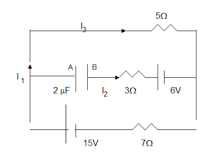# Day 121

## RC Circuit Problem

In the following example, the currents are steady in the circuit. Find I1, I2, I3 and the charge on the capacitor.• Since the capacitor has a constant charge, the current to the capacitor is zero. Therefore I2=0. Now the center wire acts as if it is missing.
• The total voltage of the circuit is now only due to the 15V battery.
• The total resistance is due to the sum of the 5Ω and 7Ω resistors, therefore it equals 12Ω (in series).
• The total current, Itot = Vtot/Rtot = 15V/12Ω = 1.25 A
• Since I2=0, I1=I3 =1.25 A (in series)
• To find the capacitor charge, find VAB by using the top (or bottom loop) and Kirchoff’s voltage rule.
• Using the top loop, going counterclockwise from A to B yields:

-5I3 + 6 + 3I2 = VAB

-5(1.25) + 6 +3 (0) = VAB

-6.25 + 6 = VAB

-0.25V = VAB

• Therefore B is at a lower potential than A and the B plate is the negative plate.
• To find the charge on the capacitor:

Q=CV = (2 x 10-6 F)(0.25V) = 5 x 10-7 C = 0.5 μC

## RC Circuit Problem #2A battery of emf of 15V is connected to three capacitors, C1 = 1.10 μF,

C2 = 2.20 μF, and C3 = 3.30 μF as shown. The resistance of the resistor is 50Ω.

(A) Find the net capacitance, Cab, between points a and b.

Since C2 and C3 are in parallel, their equivalent capacitance is simply the sum of the individual capacitances.

C2 + C3 = 2.20 x 10-6 F + 3.30 x 10-6 F = 5.50 x 10-6 F = 5.50 μF

Since C1 is in series with the other two capacitors, use the reciprocal formula:

1/Ctot = 1/C1 + 1/C2,3

1/Ctot = 1/1.10 x 10-6 F + 1/5.50 x 10-6 F

Ctot = 9.17 x 10-7 F

What is the final potential between points a and b, Vba?

Since we make the assumption that initially the capacitors are uncharged, the final potential refers to the voltage difference after the capacitors have been fully charged.

When this occurs, there is no voltage difference across the resistor because there is no current through the resistor and all of the voltage difference from the battery must now be across the charged capacitor system.

Therefore, Vba = 15V (B is at the higher potential since it is connected to the positive side of the battery and A is at the lower potential since it is connected to the negative side of the battery)

What is the charge, Q, stored in this system of capacitors?

Q = CVab = 9.17 x 10-7 F (15 V) = 1.38 x 10-5 C

What is the stored energy in the system of capacitors, U?

UC = ½ CV2 = ½ (9.17 x10-7 F)(15 V)2

UC = 1.03 x 10-4 J

What is the time constant (t) for charging the capacitors?

• Starting with an uncharged capacitor and closing the switch starts a charge flow that is time dependent
• When Q=0, V=0 and V=IinitialR (Ohm’s Law is followed)
• Any instant thereafter that charge flows, V = IR + Q/C
• When the capacitor is fully charged, V = Q/C, because I=0
• t = RC

t = RC = (50Ω)(9.17 x 10-7F) = 4.59 x 10-5 s

(source)